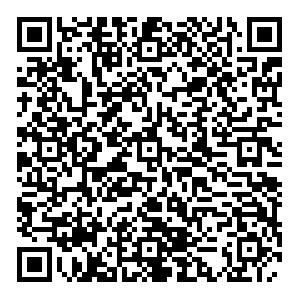# Double-heavy tetraquark states with heavy diquark-antiquark symmetry

• We calculate the masses of the $QQ\bar{q}\bar{q}$($Q=c,b$; $q=u,d,s$) tetraquark states with the aid of heavy diquark-antiquark symmetry (HDAS) and the chromomagnetic interaction (CMI) model. The masses of the highest-spin ($J=2$) tetraquarks that have only the $(QQ)_{\bar{3}_c}(\bar{q}\bar{q})_{3_c}$color structure are related with those of conventional hadrons using HDAS. Thereafter, the masses of their partner states are determined with the mass splittings in the CMI model. Our numerical results reveal that (i) the lightest $cc\bar{n}\bar{n}$($n=u,d$) is an $I(J^P)=0(1^+)$state around 3929 MeV (53 MeV above the $DD^*$threshold), and none of the double-charm tetraquarks are stable; (ii) the stable double-bottom tetraquarks are the lowest $0(1^+)$$bb\bar{n}\bar{n}$around 10488 MeV ($\approx116$MeV below the $\bar{B}\bar{B}^*$threshold) and the lowest $1/2(1^+)$$bb\bar{n}\bar{s}$around 10671 MeV ($\approx20$MeV below the $\bar{B}\bar{B}_s^*/\bar{B}_s\bar{B}^*$threshold); and (iii) the two lowest $bc\bar{n}\bar{n}$tetraquarks, namely the lowest $0(0^+)$around 7167 MeV and the lowest $0(1^+)$around 7223 MeV, are in the near-threshold states. Moreover, we discuss the constraints on the masses of double-heavy hadrons. Specifically, for the lowest nonstrange tetraquarks, we obtain $T_{cc} < 3965$MeV, $T_{bb} < 10627$MeV, and $T_{bc} < 7199$MeV.
••Get Citation
Jian-Bo Cheng, Shi-Yuan Li, Yan-Rui Liu, Zong-Guo Si and Tao Yao. Double-heavy tetraquark states with heavy diquark-antiquark symmetry[J]. Chinese Physics C.
Jian-Bo Cheng, Shi-Yuan Li, Yan-Rui Liu, Zong-Guo Si and Tao Yao. Double-heavy tetraquark states with heavy diquark-antiquark symmetry[J]. Chinese Physics C.Milestone
Article Metric

Article Views(64)
Cited by(0)
Policy on re-use
To reuse of subscription content published by CPC, the users need to request permission from CPC, unless the content was published under an Open Access license which automatically permits that type of reuse.
###### 通讯作者: 陈斌, bchen63@163.com
• 1.

沈阳化工大学材料科学与工程学院 沈阳 110142

Title:
Email:

## Double-heavy tetraquark states with heavy diquark-antiquark symmetry

• 1. School of Physics, Shandong University, Jinan, Shandong 250100, China

Abstract: We calculate the masses of the $QQ\bar{q}\bar{q}$($Q=c,b$; $q=u,d,s$) tetraquark states with the aid of heavy diquark-antiquark symmetry (HDAS) and the chromomagnetic interaction (CMI) model. The masses of the highest-spin ($J=2$) tetraquarks that have only the $(QQ)_{\bar{3}_c}(\bar{q}\bar{q})_{3_c}$color structure are related with those of conventional hadrons using HDAS. Thereafter, the masses of their partner states are determined with the mass splittings in the CMI model. Our numerical results reveal that (i) the lightest $cc\bar{n}\bar{n}$($n=u,d$) is an $I(J^P)=0(1^+)$state around 3929 MeV (53 MeV above the $DD^*$threshold), and none of the double-charm tetraquarks are stable; (ii) the stable double-bottom tetraquarks are the lowest $0(1^+)$$bb\bar{n}\bar{n}$around 10488 MeV ($\approx116$MeV below the $\bar{B}\bar{B}^*$threshold) and the lowest $1/2(1^+)$$bb\bar{n}\bar{s}$around 10671 MeV ($\approx20$MeV below the $\bar{B}\bar{B}_s^*/\bar{B}_s\bar{B}^*$threshold); and (iii) the two lowest $bc\bar{n}\bar{n}$tetraquarks, namely the lowest $0(0^+)$around 7167 MeV and the lowest $0(1^+)$around 7223 MeV, are in the near-threshold states. Moreover, we discuss the constraints on the masses of double-heavy hadrons. Specifically, for the lowest nonstrange tetraquarks, we obtain $T_{cc} < 3965$MeV, $T_{bb} < 10627$MeV, and $T_{bc} < 7199$MeV.

### HTMLII.   MODEL AND METHODIII.   ${cc\bar{n}\bar{n}}$ SPECTRUM IN TERMS OF KNOWN ${ \Xi_{cc}}$IV.   PREDICTIONS FOR OTHER DOUBLE-HEAVY TETRAQUARK STATESV.   CONSTRAINTS ON MASSES OF ${ QQq }$, $QQQ}$, AND ${QQ\bar{q}\bar{q}}$ STATESVI.   DISCUSSIONS AND SUMMARY
Reference (110)
PDF查看关注分享

Top

### 目录/DownLoad:  Full-Size Img  PowerPoint